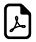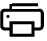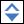# Table of Basic Math Symbols with Examples## Math Basic Symbols with Examples

SymbolSymbol NameSymbol MeaningExample+ Plus Sign addition 10 + 5 = 15
Minus Sign subtraction 10 − 9 = 8
= Equals Sign equality 6 = 5+1
> Strict Inequality greater than 55 > 14
< Strict Inequality less than 44 < 55
Inequality greater than or equal to 10 ≥ 9
Inequality less than or equal to 9 ≤ 10
( ) Parentheses calculate expression inside first 1 × (3+7) = 10
[ ] Brackets calculate expression inside first [(1+1)*(1+1)] = 4
± Plus - Minus both plus and minus operations 3 ± 4 = 7 and -1
Minus - Plus both minus and plus operations 23 = -1 and 5
* Asterisk multiplication 3 * 3 = 9
× Times Sign multiplication 5 × 5 = 25
∙  Multiplication Dot multiplication 10 ∙ 10 = 100
÷ Division Sign division 10 ÷ 5 = 2
/ Division Slash division 8 / 4 = 2
mod Modulo remainder calculation 5 mod 2 = 1
. Period decimal point 2.56 = 2+56/100
ab Power exponent 33 = 27
a^b Caret exponent 3 ^ 3 = 27
a Square Root a. a  = a 9= ±3
3a Cube Root 3a. 3a  . 3a  =a 38 = 2
4a Fourth Root 4a. 4a  . 4a  . 4a  =a 416 =±2
na N-th Root (radical)   for n=3, n8 = 2
% Percent 1% = 1/100 10% × 30 = 3
Per-Mille 1‰ = 1/1000 = 0.1% 10‰ × 30 = 0.3
ppm Per-Million 1ppm = 1/1000000 10ppm × 30 = 0.0003
ppb Per-Billion 1ppb = 1/1000000000 10ppb × 30 = 3×10-7
ppt Per-Trillion 1ppt = 10-12 10ppt × 30 = 3×10-10